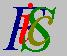Mauro Murzi's pages on Philosophy of Science - Quantum mechanics
Features of Schrödinger quantum mechanicsAbstract Complex numbers# [1. Introduction.]

## Features of Schrödinger quantum mechanics.

Schrödinger quantum mechanics is a restricted portion of quantum theory. Its main limitation is due to the fact that it is a non-relativistic theory. This is a very important limitation, because every fundamental theory must be a relativistic one. Schrödinger equations find an application only in situation in which the energy is small. Events concerning creation or annihilation of particles require a great value of the energy, and thus this type of events is out of the scope of Schrödinger quantum theory.

However, this theory gives an explanation of important facts, like the quantization of the energy levels in hydrogen atom. The indeterminacy principle is derivable from Schrödinger quantum mechanics, and this fact is of a primary interest from a philosophical analysis.

Perhaps the most important point concerning Schrödinger quantum mechanics is that its mathematical complexity is not overwhelming. A philosophical analysis of a scientific theory requires an effective comprehension of the theory, which is often very difficult due to the complexity of the required mathematical background. Schrödinger quantum mechanics requires a mathematical knowledge limited to the the first-year calculus: derivative and integral of a real function. With a little effort, derivative, integral and expansion of complex functions can be understood using the analogy with real functions.Abstract Complex numbers